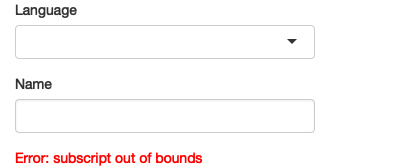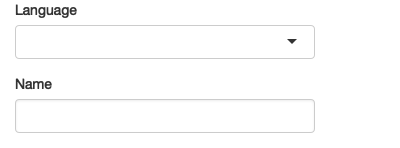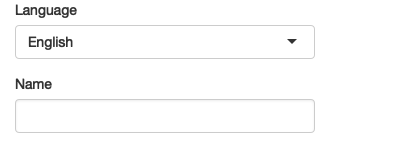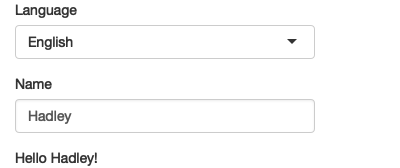# Chapter 7 User feedback

It’s important to give the user feedback as they interact with your app. Some feedback occurs naturally through outputs, but often you’ll need a side-channel to let the user know that they’ve given the app an invalid input or its taking a little while for some action to occur. In this chapter, you’ll learn how to keep the user up to date with what’s happening with validation message and notifications. Many of the techniques described here are best thought of as side-effects; they are things that happen at the end of the reactive graph.

``library(shiny)``

## 7.1 Validation

The first and most important feedback you can give to the user is that they’ve given you bad input.

It’s particularly important to think through how the user might use your app incorrectly so that you can give them informative messages in the UI, rather than allowing errors to trickle through into the R code where the error messages might not be so important.

In this section, you’ll learn about the `req()` and `validate()` functions.

### 7.1.1 Ignore missing inputs

Sometimes you don’t want to do anything until the user has performed some action. By default, a reactive will perform computation as soon as the app loads, but sometimes you want to wait until the user has provided some data.

This occurs primarily with three controls:

• `textInput()` starts off blank (`""`) so you may want to delay computation until the user has entered some text.

• In `inputSelect()` you may have provided an empty choice, `""`, to indicate no selection.

• `fileInput()` will have an empty result until the user has uploaded a file. See Section @ref(#upload) for more details.

The easiest way to fix this problem is with `req()`: it checks that the input has been set before proceeding. `req()` sends a special signal to Shiny telling it that the reactive isn’t ready yet. For example, consider the following app which will generate a greeting in English or Maori. If you run this app, you’ll see an error, as in Figure 7.1. That’s because there’s no entry in the `greetings` vector that corresponds to the choice of `""`.

``````ui <- fluidPage(
selectInput("language", "Language", choices = c("", "English", "Maori")),
textInput("name", "Name"),
textOutput("greeting")
)

server <- function(input, output, session) {
greetings <- c(
English = "Hello",
Maori = "Ki ora"
)
output\$greeting <- renderText({
paste0(greetings[[input\$language]], " ", input\$name, "!")
})
}``````Figure 7.1: The app displays an uninformation error when it is loaded because language hasn’t been selected yet

You can fix this problem by using `req()`. Now nothing will be displayed until the user has suppled values for both language and name, as shown in Figure 7.2.

``````server <- function(input, output, session) {
greetings <- c(
English = "Hello",
Maori = "Ki ora"
)
output\$greeting <- renderText({
req(input\$language, input\$name)
paste0(greetings[[input\$language]], " ", input\$name, "!")
})
}``````Figure 7.2: By using `req()`, the output is only shown once both language and name have been supplied

`req()` is designed so that `req(input\$x)` should just work regardless of what type of input `x` is: if you’re interested you can read about the details in `?isTruthy`. You can also use `req()` with your own logical statement if needed. For example, `req(input\$a > 0)` will only trigger computation when a is greater than 0.

In certain cases, you might want to leave the last output up. For example, imagine you allow the user to type the name of a dataset. As they’re typing, reactives will be firing, and it’d be nice to only change the output once they’ve typed a complete name.

``````ui <- fluidPage(
textInput("dataset", "Dataset name"),
tableOutput("data")
)
server <- function(input, output, session) {
output\$data <- renderTable({
req(input\$dataset)
req(exists(input\$dataset, "package:datasets"), cancelOutput = TRUE)
})
}``````

Also note that I’ve used `req()` twice; the first time prevents `exists("")` being called, which triggers an error.

`req()` works by signalling a special condition (condition is the term that refers jointly to errors, warnings, and messages). This special condition causes all downstream reactives and outputs to stop executing. Technically, it leaves any downstream reactive consumers in an invalidated state. We’ll come back to this terminology in Chapter @ref{reactive-components}.

### 7.1.2 Validate input

If you want to give additional feedback to the user, a great way to do so is with the shinyFeedback package. There are two steps to use it. First, you add `useShinyFeedback()` to the ui:

``````ui <- fluidPage(
shinyFeedback::useShinyFeedback(),

textInput("dataset", "Dataset name"),
tableOutput("data")
)``````

Then you call `feedbackDanger()` or `feedbackWarning()` in the server function. Since the logic is starting to get complicated, I’ve pulled out the validation into a reactive. The sequence of operations is basically the same as before except now that I pull out `exist` into a separate variable and use it for both `feedbackDanger()` and `req()`.

``````server <- function(input, output, session) {
data <- reactive({
req(input\$dataset)

exists <- exists(input\$dataset, "package:datasets")
shinyFeedback::feedbackDanger("dataset", !exists, "Unknown dataset")
req(exists, cancelOutput = TRUE)

get(input\$dataset, "package:datasets")
})

output\$data <- renderTable({
})
}``````

Note that `feedbackDanger()` is parameterised in the opposite direction to `req()` so it gets a `!`.

### 7.1.3 Validate output

Sometimes the problem is not related to a single input, but is related to an invalid combination of inputs. In this case, rather than putting the error message next to the input, it might make more sense to put it in the output. You can do so with a tool built into shiny: `validate()`.

``````ui <- fluidPage(
numericInput("x", "x", value = 0),
selectInput("trans", "transformation", choices = c("square", "log", "square-root")),
textOutput("out")
)

server <- function(input, output, server) {
output\$out <- renderText({
if (input\$x < 0 && input\$trans %in% c("log", "square-root")) {
validate("x can not be negative for this transformation")
}

switch(input\$trans,
square = input\$x ^ 2,
"square-root" = sqrt(input\$x),
log = log(input\$x)
)
})
}``````

### 7.2.1 Progress

• Can divide the task into units that take roughly the same amount of time.
• Can divide the task into discrete steps

Progress bar. Simplest way is to use `withProgress()`

``````ui <- fluidPage(
numericInput("steps", "How many steps?", 5),
actionButton("go", "go")
)
server <- function(input, output, session) {
observeEvent(input\$go, {
withProgress(message = "Hi!", {
for (i in seq_len(input\$steps)) {
Sys.sleep(0.1)
setProgress(i / input\$steps, message = NULL)
}
})
})
}``````

If you need more control, see the details in `?Progress` and https://shiny.rstudio.com/articles/progress.html.

With progress package - i.e. forwarding the conditions it generates to `withProgress()`. Should be able to make simple wrapper.

### 7.2.2 Message

If you don’t know how long some code will take, a better approach is to use notifications.

``````server <- function(input, output, session) {
data <- reactive({

})
}``````

Two important ideas here:

• We captured the notification id created by `showNotification()`.

• We use `on.exit()` to automatically cancel the notification when the reactive complete, regardless of whether it returns a value or throws an error.

Also note the use of `duration = NULL` and `closeButton = FALSE` that makes the notification most suitable for this task, ensuring that is stays visibile until the data loading is done.

(For this specific case you should also look at `data.table::fread()` and `vroom::vroom()` to read in the file; they can be orders of magnitude faster than `read.csv()`. And pointer to chapter about performance/promises/future)

You can slightly extend this approach to send multiple notifications if there are discrete steps (where you also don’t know how long they’ll take)

``````server <- function(input, output, session) {
data <- reactive({